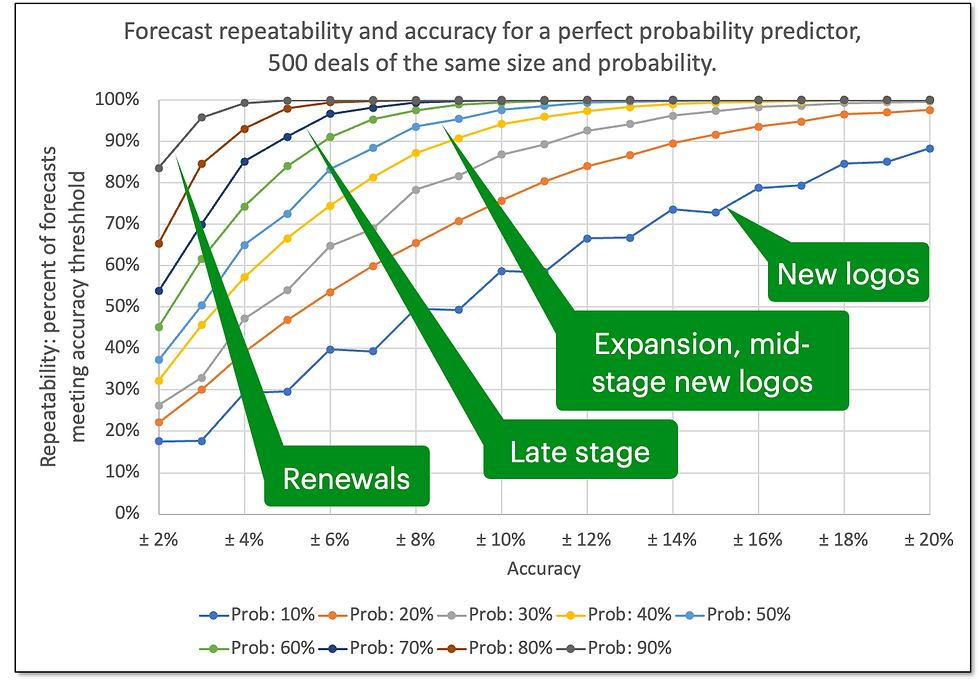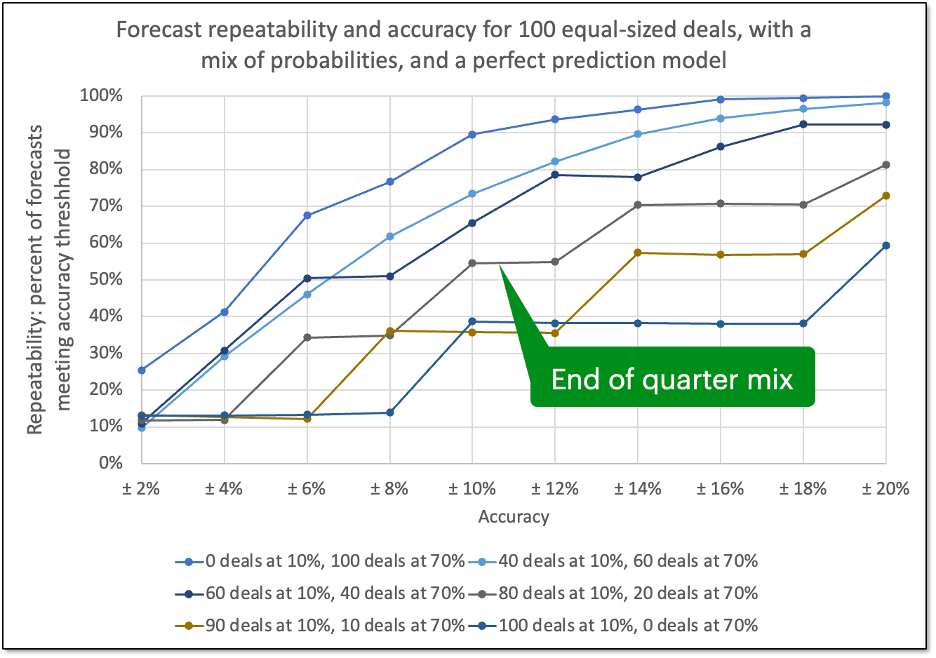top of page
Search

# Forecast Accuracy and Repeatability

Updated: 17 hours ago

Last month I blogged that All forecasts are wrong. Some are useful. The article underscored that forecast accuracy is overhyped—a distraction—and that the focus is better placed on insights a forecast model can offer. A good model helps you focus on the right things at the right time—so you can sell more.Some forecast accuracy claims. These are are unachievable and distract from what really matters.

In that blog I mentioned that it is not possible to get 2% accuracy reliably. If you make enough forecasts, eventually you will nail one. But repeatedly nailing forecasts throughout the quarter and every quarter is a lot harder. Accuracy claims are meaningless if they don’t also state repeatability.

To illustrate how difficult repeatable 2% accuracy is, I cited an (unrealistically optimistic) example which would yield ± 2% accuracy, 80% of the time. (We consider 80% repeatability to be a good standard.) This is truly outstanding performance. But how realistic is this?

To meet that performance, you would need:

1.) 500 deals in your pipeline

2.) Each of the same size

3.) Each with 90% probability of closing

4.) A forecasting model that is a perfect probability predictor 

5.) No new deals enter the sales funnel after the forecast is made. (For the balance of this discussion, we will ignore this effect.)

Not very realistic. Real-world conditions make the accuracy and repeatability much worse.

There is an inverse relationship between accuracy and repeatability. Repeatability here means the percent of forecasts that fall within the specified accuracy range of actual sales.

Every forecast has an associated curve representing "frontier" of this tradeoff—the highest repeatability for a given set of conditions. We wanted to understand the shape of this relationship and what is possible.Figure 1

We used Monte Carlo simulations to explore these questions. The charts here show the effects (using 10,000 simulations in each case) of varying the number of deals and probabilities, and otherwise conforming to the constraints 1 – 5 above. To follow real-world conditions more closely, we also explored the effects of a mix of different probabilities.

The results? Unless your business is almost all high probability deals (like subscription renewals), chasing forecast accuracy will have limited return. Instead, you should focus on what your forecast model tells you about how to optimize and sell more.

Figure 2 shows the simulated accuracy and repeatability tradeoff for 500 same size and probability deals.  You can see the data point we cited in our example—top line, left-most datapoint. If you had 500 equal sized deals, of 90% probability, a perfect probability predictor, and no new deals entering the funnel, you could get within 2% accuracy 84% of the time. Each of the other lines represents the tradeoff between accuracy and repeatability for sets of 500 deals of different probabilities. The callouts underscore the types of deals represented by some of the lines.

If your business were all renewals, then achieving high accuracy and repeatability would be possible. To the extent that you are forecasting renewals, it forms a reliable base of business that reduces the overall volatility of a forecast that also includes lower probability deals.Figure 2. A perfect probability predictor, 500 same size and probability deals.

Figure 3 shows same repeatability-accuracy tradeoff for a smaller sales funnel of 100 deals.  With fewer deals in your funnel, repeatability is dramatically diminished for the same accuracy. The 2% accuracy is only possible less than 40% of the time for the same 90% probability deals. And 20% accuracy 80% of the time, is only possible if you have greater than 30% probability deals.Figure 3. A perfect probability predictor, 100 same size and probability deals.

Figure 4 (20 deals) shows how impractical it is (under these idealized conditions) to get even 20% accuracy with high reliably for small funnels.Figure 4. A perfect probability predictor, 20 same size and probability deals.

None of these are real-world scenarios. But they highlight the upper bounds of what is possible.

Simulating real world scenarios is difficult because in addition to the number of deals, your results would depend on your mix of deal probabilities, their sizes, and how good your predictive model is.

Let’s explore the effect of having a mix of deal probabilities. A rough way to model this is to simulate the effects of varying a mix of low probability deals (for which you would have the lowest accuracy forecasts) and high probability deals (producing the highest accuracy forecasts).

Figure 5 shows this for a business with 500 deals in their pipeline and various mixes of 10% and 70% probability deals (think of them as early-stage new logo opportunities and late-stage deals). Real distributions of probabilities are not bi-modal like that. We are simplifying to see what we can learn.

The top line (all 70% deals) is the same as the 70% line in Figure 1. The other lines show the effects of blending in a mix of 10% probability deals.

A forecast for a business with 500 (equal sized) deals at the start of their quarter might be approximated by one of those lower lines in Figure 5—some high probability deals and a large group of low probability deals. With the mix indicated by the callout, getting 80% repeatability requires relaxing the accuracy to considerably, to greater than 16%. Accuracies of 2% to 6% have very low repeatability.Figure 5. A perfect probability predictor, 500 same size deals with a mix of probabilities.

With 100 deals in your pipeline (Figure 6), it is impossible to achieve 80% repeatability, even with 20% accuracy for our start of quarter mix (bottom line). At the end of a quarter, businesses have narrowed down their pipeline. A business that started the quarter with 500 deals might have 100 active deals remaining. Their mix might look something like one of the middle lines of Figure 6. The outcome on this smaller funnel is more volatile than the forecast at the start of the quarter. But the deals won prior to that point in the quarter provide a cushion reducing the forecast volatility as a percent of the total sales for the quarter.Figure 6. A perfect probability predictor, 100 same size deals with a mix of probabilities.

Businesses with smaller pipelines might experience something similar to Figure 7 (20 deals). Sales pipelines of this size are hard to predict reliably and accurately.Figure 7. A perfect probability predictor, 20 same size deals with a mix of probabilities.

Summary

These studies show that—even under the most favorable conditions—forecast accuracy of better than 10% and 80% repeatability is unrealistic. While high accuracy is desirable, chasing this is not likely to be fruitful. You may achieve high accuracy in one forecast period, it will likely not be repeatable.

Given these inherent limitations, we suggest you think about how your forecasts can help you optimize sales. Read our blog on this: All forecasts are wrong. Some are useful.

Notes:

 We are making probabilistic calls on individual deals to produce a weighted forecast. In contrast, one could make binary (win/lose) calls on deals. With enough deals, the weighted approach provides better forecasts. So, when we say that the forecasting model is a “perfect probability predictor,” we mean that on average, if the model predicts say a 70% chance of closing for a group of deals, that 70% of those will be won.

 That jagged 10% deal probability line is a real. Repeatability is constrained by integer wins. E.g., 500 deals at 10% probability would mean you would forecast 50 wins. And 2% error means your actual sales would need to be between 49 and 51 (inclusive). A 3% error would mean that your actuals would be between 48.5 and 51.5 deals. Since you can’t win fractional deals, the 3% error is the same as the 2% error.

 Surprisingly, the some of the lines of Figure 2 and Figure 3 show higher repeatability for lower probability deals. This non-linearity is a real quirk due to the nature of the number of deals and probabilities. E.g., with 20 deals at 10% probability would expect 2 deals. The range of 20% accuracy is 1.6 to 2.4 deals. Since outcomes are only integer values, there is no distinction between the 20% accuracy and 2% accuracy. The same holds for 20% probability deals. And the 20% probability point is less likely to occur because the distribution of outcomes if you expect 2 deals (with 10% probability) is more closely packed than if you expect 4 deals (with 20% probabilities).

See All
bottom of page